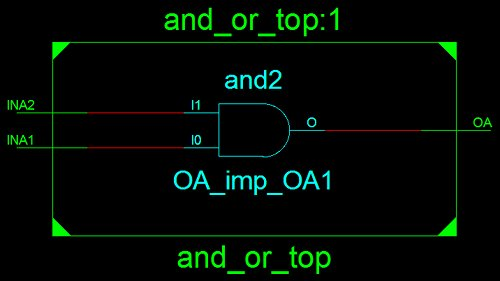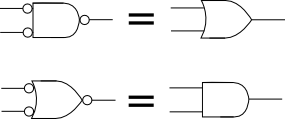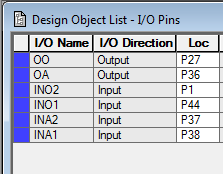Starting Electronics needs your help! Please make a donation to help cover our hosting and other costs. Click the donate button to send a donation of any amount.# Tutorial 2: AND Gates, OR Gates and Signals in VHDL

Created on: 7 December 2012

In this second tutorial of the VHDL course, we look at two basic logic gates, namely the AND gate and the OR gate. We also look at signals in VHDL.

An interesting problem can occur in a logic design that turns an AND gate into an OR gate. This same problem also turns an OR gate into an AND gate. These problems occur because of the external wiring of the logic system when it inverts inputs and outputs. VHDL signals are used to compensate for this problem in the CPLD circuit used in this tutorial.

In the VHDL code in this tutorial, you will see the name and_or which is the name of the Xilinx project used with this tutorial. A single project was created to demonstrate both the AND and OR gates. This code is separated out in the first listings below to help explain each gate separately.

## AND Gates in VHDL

The VHDL for a two input AND gate is shown below:

```library IEEE;
use IEEE.STD_LOGIC_1164.ALL;

entity and_or_top is
Port ( INA1 : in  STD_LOGIC;    -- AND gate input
INA2 : in  STD_LOGIC;    -- AND gate input
OA   : out STD_LOGIC;    -- AND gate output
end and_or_top;

architecture Behavioral of and_or_top is
begin
OA <= INA1 and INA2;    -- 2 input AND gate
end Behavioral;
```

The VHDL and keyword is used to logically AND the INA1 and INA2 inputs together. The result of the AND operation is put on the OA output by using the VHDL <= operator.

This is the equivalent gate described by the above code:Schematic Symbol of the AND Gate

## OR Gates in VHDL

This is the VHDL code for a two input OR gate:

```library IEEE;
use IEEE.STD_LOGIC_1164.ALL;

entity and_or_top is
Port ( INO1 : in  STD_LOGIC;    -- OR gate input
INO2 : in  STD_LOGIC;    -- OR gate input
OO : out  STD_LOGIC);    -- OR gate output
end and_or_top;

architecture Behavioral of and_or_top is
begin
OO <= INO1 or INO2;    -- 2 input OR gate
end Behavioral;
```

This code works the same as the AND example, but this time the VHDL or operator is used to perform a logic OR operation between the two inputs.

This is the equivalent gate described by the above code:Schematic Symbol of the OR Gate

## Input and Output Inversion Problem

A problem occurs on the home made Xilinx CPLD board when the above AND and OR gates are implemented and the switches from the switch bank are used as inputs to the gates and the LEDs are used as outputs from the gates. This problem will occur on other boards that have inputs and outputs configured the same way as the CPLD board.

The symptoms of the problem is that the AND gate behaves like an OR gate; the OR gate behaves like an AND gate.

The reason that this problem is occurring is because of the way the switches and LEDs are wired on the CPLD board. The switches from the switch bank change the inputs to the CPLD from high to low when closed. The LEDs switch on when a low is output from the CPLD to the LEDs.

What this wiring is doing is effectively inverting the inputs to the CPLD and output from the CPLD: when the switch is closed, we expect to be putting a logic 1 on the CPLD pin, but we are putting a logic 0 on the CPLD pin instead; when the output from a CPLD pin is set to a logic 1 level, we are actually switching the LED off rather than on.

This diagram shows what effectively is happening:When Inputs and Outputs of the Gates on the Left are Inverted,
they Effectively Become the Gates Shown on the Right

This behaviour of the inverting inputs and outputs can be compensated for in the VHDL code.

## Compensating for the Inverting Switches and LEDs

We can use signals in VHDL to compensate for the inverting switches and LEDs. Signals can be thought of as being equivalent to variables in computer programming languages. Signals are internal to the CPLD (or FPGA).

The idea is to create a signal for each input and each output of the gate. The input values on the gates can now be read from the CPLD pins, then inverted and assigned to the signals. The outputs of the gates can be assigned to signals and then inverted before sending the gate output to CPLD output pin. This compensates for the inverting switches and LEDs by inverting their values so than an on switch will now read as logic 1; a LED will be switched on by a logic 1.

The VHDL code for both the AND gate and OR gate will now look as follows:

```library IEEE;
use IEEE.STD_LOGIC_1164.ALL;

entity and_or_top is
Port ( INA1 : in  STD_LOGIC;	-- input of AND gate
INA2 : in  STD_LOGIC;	-- input of AND gate
OA   : out STD_LOGIC;	-- output of AND gate

INO1 : in  STD_LOGIC;	-- input of OR gate
INO2 : in  STD_LOGIC;	-- input of OR gate
OO   : out STD_LOGIC);	-- output of OR gate
end and_or_top;

architecture Behavioral of and_or_top is
signal A1 : STD_LOGIC;	-- internal input of AND gate
signal A2 : STD_LOGIC;	-- internal input of AND gate
signal X1 : STD_LOGIC;	-- internal output of AND gate
signal B1 : STD_LOGIC;	-- internal input of OR gate
signal B2 : STD_LOGIC;	-- internal input of OR gate
signal Y1 : STD_LOGIC;	-- internal output of OR gate
begin

-- old AND gate code commented out
--OA <= INA1 and INA2;

X1 <= A1 and A2;   -- new AND gate using the inverted internal signals

-- old OR gate commented out
--OO <= INO1 or INO2;

Y1 <= B1 or B2;    -- new OR gate using the inverted signals

-- compensation for inverting inputs and outputs
A1 <= not INA1;    -- invert the external input pin and assign it to AND gate input A1
A2 <= not INA2;    -- invert pin INA2, assign to A2 AND gate input
OA <= not X1;      -- invert the output of the AND gate, assign to pin OA
B1 <= not INO1;    -- etc.
B2 <= not INO2;
OO <= not Y1;
end Behavioral;
```

In the above code, first note that signals are declared between the architecture and begin statements.

Effectively, the compensation code can now be ignored. The AND gate code is now this single line:

```X1 <= A1 and A2;
```

The OR gate code is now this:

```Y1 <= B1 or B2;
```

A1, A2, B1 and B2 can be thought of as inputs connected directly to the switch bank. When a switch is switched on, it can now be thought of as producing a logic 1 on the CPLD pin.

X1 and Y1 can be thought of as outputs connecting directly to the LEDs. To switch an LED on, a logic 1 can be sent to X1 or Y1.

The above assumes that the UCF file has been configured to wire the switches and LEDs to the gate inputs and outputs.

### Concurrency

The above code demonstrates concurrency in VHDL. The statements do not run sequentially from top to bottom, but rather in parallel. The compensation code could therefore be put above the gate implementation, or below it.

The AND and OR gates also run in parallel. The code does not first run the AND gate part and then the OR gate part.

This video shows the above code running on the home made Xilinx CPLD board:

Can't see the video? View on YouTube →

## Creating the Project with the Xilinx Tools

The project can be created using the above code and then using Xilinx PACE to create the UCF file to connect the inputs of the gates to switches on the switch bank and outputs to the LEDs.

The pins used on the home made CPLD board for this tutorial are shown below in Xilinx PACE.Pin Assignment in Xilinx PACE

This connects the outer two switches of the switch bank to one gate and the outer two switches on the opposite side of the switch bank to the other gate.

### Source Code

The project created for this tutorial is called and_or and the VHDL file is called and_or_top.vhd.

VHDL file, UCF file and JED file: and_or_vhd_ucf_jed.zip (5.9kB)

Entire Xilinx project: and_or.zip (690.6kB)

## Multiple Input AND and OR Gates

A gate with any number of inputs can be created by stringing more inputs together with AND or OR statements. The following code shows a three input AND gate:

```X <= A1 and A2 and A3;
```

Don't forget to add the extra inputs to the Port in the entity part of the code and then assign a pin on the CPLD using Xilinx PACE.

Books that may interest you: